# Antenna Gain

Antenna gain is associated with the ability of the antenna or antenna system to direct the radiated power in a specific direction or conversely, absorb the power efficiently coming from a particular direction. Gain is regarded as the fundamental quantity of antenna as it relates to the efficiency of the antenna system.

The gain of the antenna is highly associated with the directivity. While the directivity shows complete dependency on the radiation pattern offered by the antenna.

Before exploring more details about antenna gain let us first have a look at the contents to be discussed here.

## Content: Antenna Gain

### What is an Antenna?

An antenna is regarded as a fundamental unit of wireless communication and is basically a transducer that changes one form of energy into another.

The antenna is designed to convert radio frequency electrical signal into the electromagnetic wave and the emitted EMW is further transmitted from an end to the other with the speed of light. This implies that transmitting the signal in a form of electromagnetic wave facilitates wireless communication with great speed and less distortion.

This is the reason antennas are used for transmission. However, not only transmission but the emitted electromagnetic wave is received at the other end by an antenna unit.

Thus, antenna facilitates easy signal transmission in wireless communication applications.

## Gain of Antenna

Antennas are said to be passive devices. This is so because we know that active elements are the ones that generate a signal for their own operation.

While we have recently discussed that the antenna changes the applied electrical signal into an electromagnetic wave. This means an external signal is required to be applied at the input in order to convert it into EMW.

Hence antennas are regarded as passive elements. And due to this reason, shows variation and is not same always.

The antenna gain is regarded as a relative terminology that is determined by comparing the actual antenna with the reference one.

### Antenna Gain Measurement

In the previous section, we have seen that the gain of the antenna is determined by making a comparison of the subject antenna with the reference antenna.

So, basically for measuring the gain of the antenna, a reference antenna must be considered at the initial level.

Generally, a hypothetical lossless isotropic antenna that is known to radiate equally in all the directions is used as a reference antenna. Although in some cases half-wave dipole antenna is also used as a reference, the isotropic radiator is mostly used.

The antenna gain is specified by direction in which most of the available power is radiated.

So, the antenna gain is defined as the ratio of maximal radiation intensity in a specific direction by the subject antenna to the maximal radiation intensity in the same direction by the reference antenna, keeping the input signal identical.

Hence, symbolically the gain of the antenna is given as:Where the effect of losses is taken into consideration for both the antennas.

The isotropic radiator is omnidirectional in nature thus is said to possess 100% efficiency.

Thus, in this case, the gain of the antenna is denoted as G0 which corresponds to the gain w.r.t isotropic antenna.

So,Thus, we can write it as: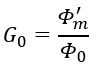: Φmdenotes the maximum radiation obtained from subject antenna and

Φ0 represents the maximum radiation achieved from the isotropic antenna.

It is to be noted here that when half-wave dipole antenna is considered as the reference antenna then the gain will vary.

We have already discussed that gain of the antenna corresponds to the amount of energy concentration. Thus, the antenna that possesses high gain, indicates a narrow beamwidth.

The gain offered by the antenna is very much related to directivity. This is so because when an isotropic antenna is considered as the reference one then antenna gain is equivalent to directivity as in this case the provided efficiency is 100%.

This can be stated in a way that, in the case of lossless antennas, the gain and directivity are the same otherwise not. So, in the case of 100 percent efficiency, the gain and directivity are regarded as the interchangeable terms.

Till now we have seen that gain of the antenna is generally characterized by the direction in which the radiation intensity is maximum. However, to represent the antenna gain in a specific direction where the radiation intensity is not maximum, then the angle Φ of that particular direction is used while representation.

Hence, symbolically,Otherwise,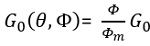: angle θ is the angle of colatitude while angle Φ is the polar angle.

Here, Φm is maximum radiation intensity and Φ is the radiation intensity along θ and Φ.

#### Gain of Receiving Antenna

Basically, a practical antenna under operation concentrates more power in a particular direction than the other. This shows the direction of maximum radiation for that particular antenna and so the field in that direction is higher than the field of the isotropic radiator radiating same total power.

This is the reason; the directivity is associated with the shape of the radiation pattern. As against, in case of gain, the rise in the strength of the signal in one particular direction by practical antenna corresponds to its gain in that direction.

So, the gain of the receiving antenna according to the received signal power is given as: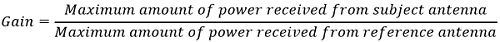Hence,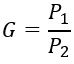### Directive Gain

It is known to us that practically antennas while radiating preferably transmit the radiated energy more in a certain direction and less in other directions.

So, the level at which the practical antenna allows the concentration of the energy relative to the direction of the standard antenna is called directive gain.

Thus, it is defined as the ratio of radiation intensity in a direction to the power radiated by the antenna on an average. It is also a function of the angle (θ and Φ) in which the radiation is considered.

It is given as:Thus, expressed as:More simply,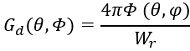The directive gain is not associated with supplied input power or the losses associated.

### Power Gain

While discussing directive gain, we have considered that both the antennas are radiating the same amount of total power.

However, while discussing the power gain of the antenna, the power density of the subject antenna and the isotropic antenna is compared as in the same input power is provided to both.

Thus,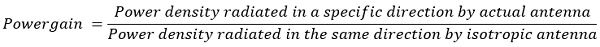It is to be noted here that directive gain and power gain are somewhat same where the only difference is power gain considers the antenna losses associated with it.

Hence, the relation between the two is given as:: η signifies the efficiency whose value lies between 0 and 1.